千次阅读 2019-01-18 14:11:00
webrtc VAD 算法 简介 一，K-means 聚类 二，EM估计 三，GMM 高斯混合模型 四，webrtc 中的 VAD 实现 五，参考资料：
webrtc VAD 算法
简介
一，K-means 聚类
K-means 属于传统机器学习中的无监督学习，体现了聚类思想，他可以对没有标签的数据进行分类，聚类过程主要分两步：
K:描述了簇的数量，也就是应当聚合成的几何数；means:均值求解，确定已分类数据的聚类中心(Cluster Centroid)位置；
具体实现步骤： (1)根据设定的聚类数 K ，随机地选择 K 个聚类中心(Cluster Centroid)，这里要注意聚类中心(Cluster Centroid)的初始值(就是图中X的位置)会影响最终的分类结果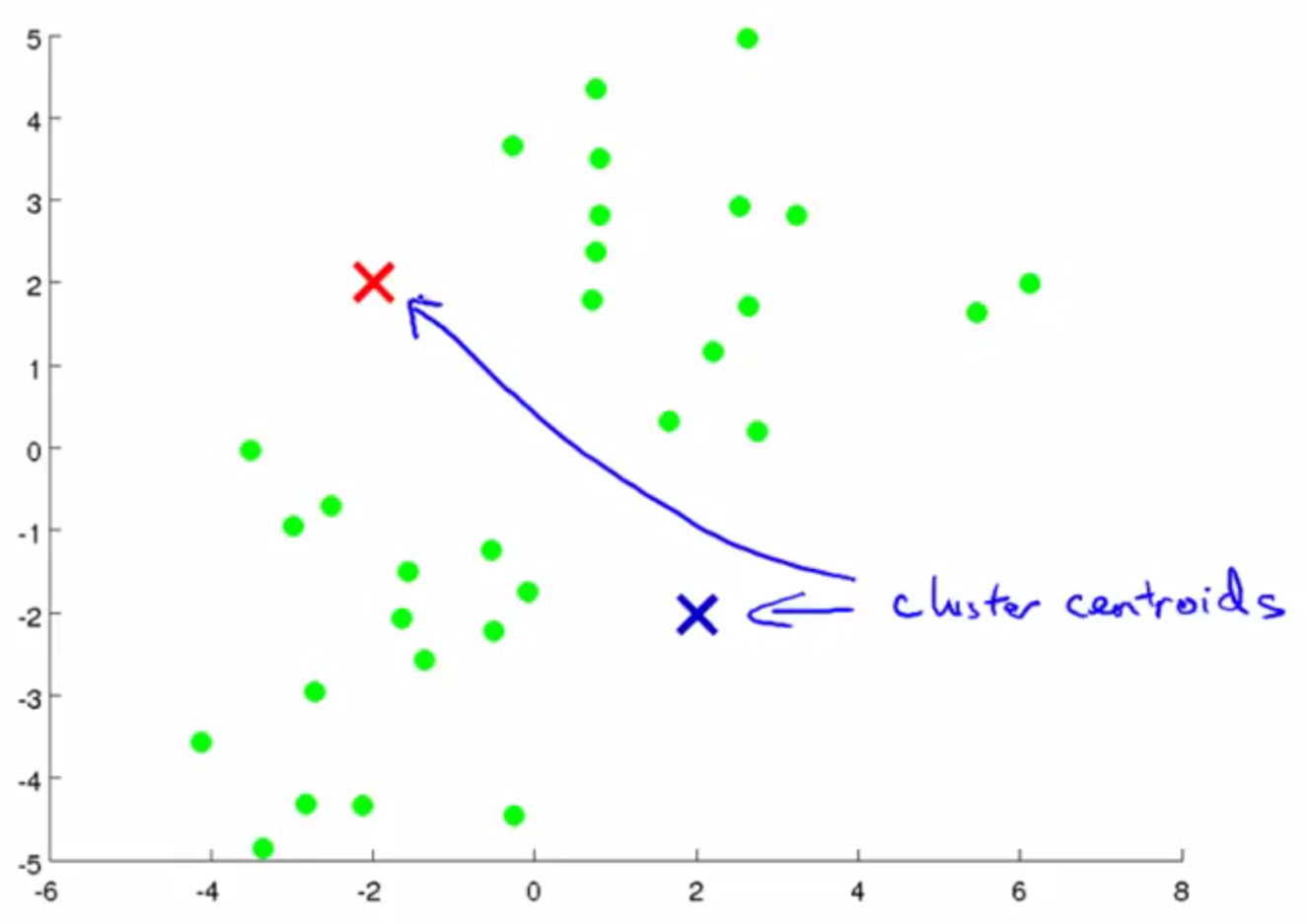(2)评估各个样本到聚类中心的距离，如果样本距离第 i 个聚类中心更近，则认为其属于第 i 簇,欧式距离：

d

=

(

x

1

−

x

2

)

2

+

(

y

1

−

y

2

)

2

d=\sqrt{(x_{1}-x_{2})^2+(y_{1}-y_{2})^2}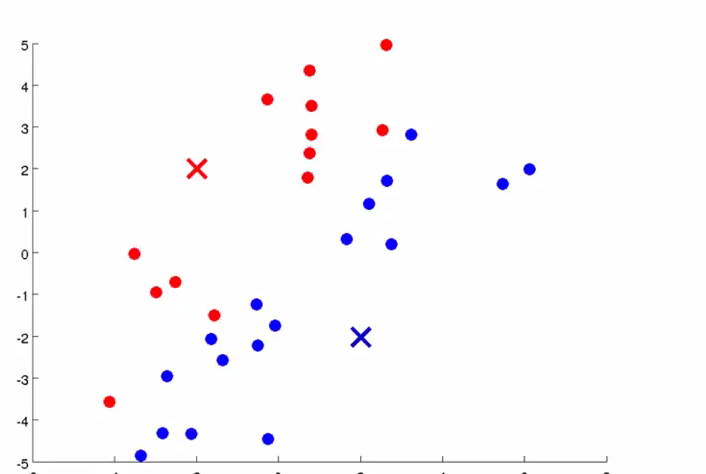(3)计算每个簇中样本的平均（Mean）位置，将聚类中心移动至该位置，实际就是求每个簇的数据在x轴和y轴上的平均值，其中μi是簇K_{i}的均值向量，有时也称为质心，表达式为：

u

i

=

1

∣

K

i

∣

∑

x

∈

K

i

x

u_{i}=\frac{1}{|K_{i}|}\sum_{x\in K_{i}}^{}x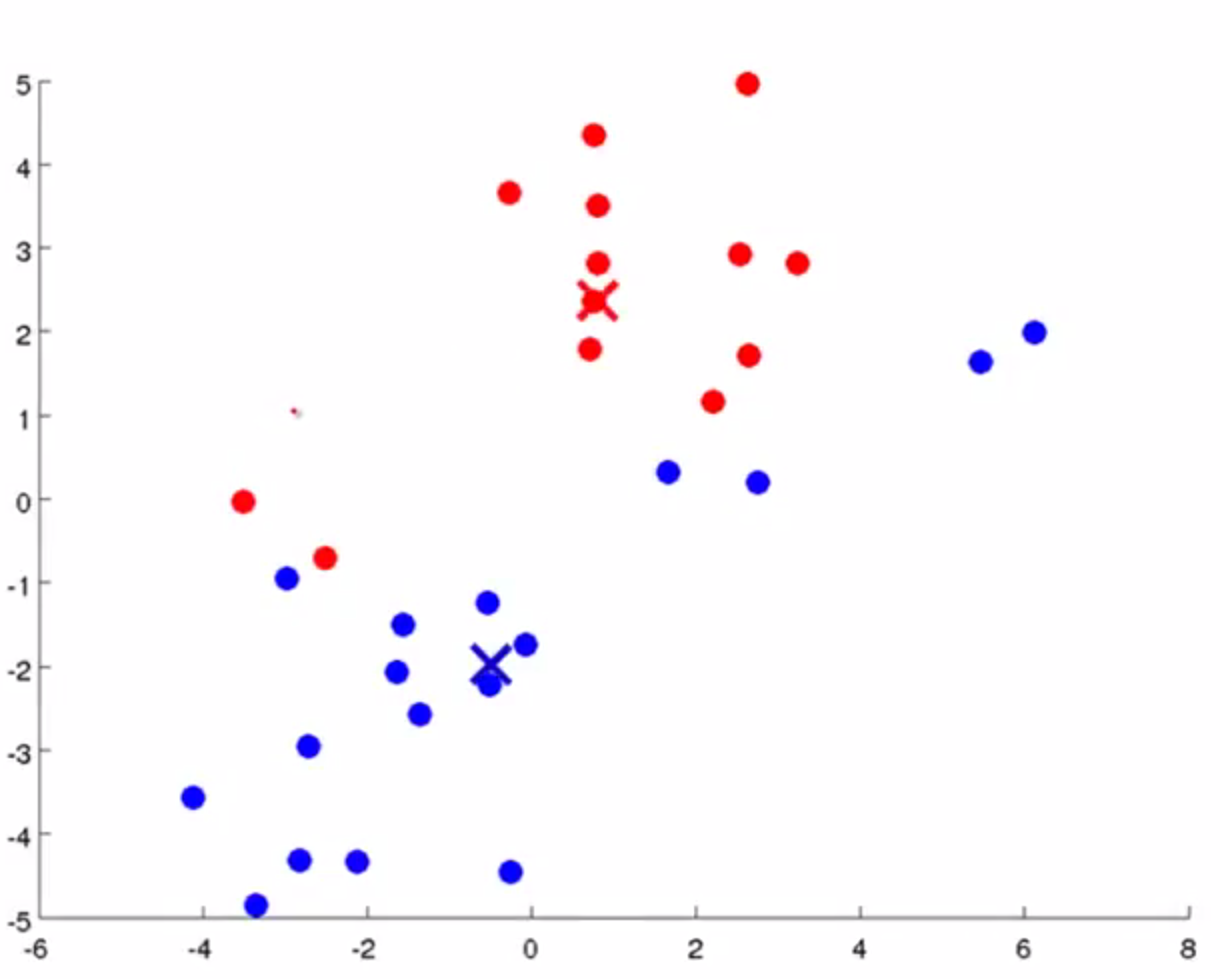重复以上(2),(3)步骤直至各个聚类中心的位置不再发生改变(这个过程一定会收敛，证明这里就不介绍了，主要理解聚类的思想)
K-means 演示实例（需要翻墙）
二，EM估计
1，似然函数和极大似然估计
硬币结果统计1正正反正反3正-2反
如上图所示，如果我们知道这个硬币正面朝上的概率是0.5，那么抛5次有3次正面朝上的概率是0.5x0.5x0.5=0.125（这个是概率），如果我们不知道这个硬币正面朝上的概率，那么抛5次有3次正面朝上，现在求这个硬币正面朝上的概率是3/5=0.6(这个就是似然)，似然和概率都是大概的意思，但是在统计学上是不同的。 现在考虑一个问题，上一步求出的硬币正面朝上的概率为什么是3/5=0.6，为什么正面朝上的次数除以总次数就是硬币正面朝上的概率，其实这个就是用极大似然估计推导出来的，下面我们就看一下这个3/5=0.6的由来。
已知硬币连续抛5次，3正2反，求硬币正面朝上的概率，假设正面朝上的概率为

θ

\theta

,似然函数为：

L

(

θ

)

=

L

(

x

1

,

.

.

.

x

n

;

θ

)

=

∏

i

=

1

n

p

(

x

i

;

θ

)

,

θ

∈

Θ

L(\theta)=L(x_{1},...x_{n};\theta)= \prod_{i=1}^{n}p(x_{i};\theta),\theta\in\Theta

在我们这个例子中似然函数为：

L

(

θ

)

=

∏

i

=

1

3

θ

∏

i

=

1

2

(

1

−

θ

)

L(\theta)=\prod_{i=1}^{3}\theta\prod_{i=1}^{2}(1-\theta)

极大似然估计就是求似然函数

L

(

θ

)

L(\theta)

最大时，

θ

\theta

的取值，这就转化成一个纯数学问题，求导，令导数等于0，就可以得到

θ

\theta

的值，为了便于分析，还可以定义对数似然函数，将其变成连加的：

H

(

θ

)

=

l

n

L

(

θ

)

=

l

n

∏

i

=

1

n

p

(

x

i

;

θ

)

=

∑

i

=

1

n

l

n

p

(

x

i

;

θ

)

,

θ

∈

Θ

H(\theta)=lnL(\theta)=ln\prod_{i=1}^{n}p(x_{i};\theta)=\sum_{i=1}^{n}lnp(x_{i};\theta),\theta\in\Theta

在我们这个例子中对数似然函数为：

H

(

θ

)

=

l

n

L

(

θ

)

=

l

n

∏

i

=

1

3

θ

+

l

n

∏

i

=

1

2

(

1

−

θ

)

=

∑

i

=

1

3

l

n

θ

+

∑

i

=

1

2

l

n

(

1

−

θ

)

H(\theta)=lnL(\theta)=ln\prod_{i=1}^{3}\theta+ ln\prod_{i=1}^{2}(1-\theta)=\sum_{i=1}^{3}ln\theta+\sum_{i=1}^{2}ln(1-\theta)

我们对

H

(

θ

)

H(\theta)

求导，并令导数等于0，求解

θ

\theta

:

H

(

θ

)

=

∑

i

=

1

3

l

n

θ

+

∑

i

=

1

2

l

n

(

1

−

θ

)

H(\theta)=\sum_{i=1}^{3}ln\theta+\sum_{i=1}^{2}ln(1-\theta)

H

(

θ

)

=

3

l

n

θ

+

2

l

n

(

1

−

θ

)

H(\theta)=3ln\theta+2ln(1-\theta)

H

(

θ

)

′

=

3

θ

+

2

1

−

θ

×

−

1

{H(\theta )}' =\frac{3}{\theta}+\frac{2}{1-\theta}\times-1

H

(

θ

)

′

=

3

(

1

−

θ

)

θ

(

1

−

θ

)

+

−

2

θ

(

1

−

θ

)

θ

{H(\theta )}'=\frac{3(1-\theta)}{\theta(1-\theta)}+\frac{-2\theta}{(1-\theta)\theta}

H

(

θ

)

′

=

3

−

5

θ

(

1

−

θ

)

θ

{H(\theta )}'=\frac{3-5\theta}{(1-\theta)\theta}

令

H

(

θ

)

′

=

0

{H(\theta )}'=0

，得：

3

−

5

θ

(

1

−

θ

)

θ

=

0

\frac{3-5\theta}{(1-\theta)\theta}=0

，所以

3

−

5

θ

=

0

3-5\theta=0

, 最终

θ

=

3

/

5

=

0.6

\theta=3/5=0.6

。  这就是极大似然估计，总结一下计算步骤： （1）写出似然函数； （2）对似然函数取对数，并整理； （3）求导数，令导数为0，得到似然方程； （4）解似然方程，得到的参数即为所求；
2，EM估计
通过一个简单的例子来了解EM算法，假设现在有两枚硬币1和2，,随机抛掷后正面朝上概率分别为P1，P2。为了估计这两个概率，做实验，每次取一枚硬币，连掷5下，记录下结果，如下：
硬币结果统计1正正反正反3正-2反2正反反正反2正-3反1正反正正反3正-2反
根据极大似然估计，我们很容易计算出p1和p2 p1=(3+3)/10 = 0.6 p2=2/5 = 0.4现在加大难度，还是求两枚硬币正面朝上的概率p1,p2，如下图所示：
硬币结果统计未知正正反正反3正-2反未知正反反正反2正-3反未知正反正正反3正-2反
现在引入了未知变量，如何求p1,p2呢？假设未知变量是k，它是个3维向量[k1,k2,k3]，要求k，我们需要知道p1，p2，要求p1,p2，又要知道k，怎么办呢？要解决这个问题就要用到EM估计。  首先我们假设一个p1,p2的值，然后用这个假设的值去求k，有了k之后，我们在利用极大似然估计去求p1和p2，然后看一下求出的p1和p2，与我们刚才假设的值是否相等，如果不相等，就用这个p1和p2去求k，在用k，求出一个新的p1和p2，一直迭代下去，直到求出的p1和p2与上一次求出的p1和p2相等，这个时候的p1和p2就是我们要求的答案了，这个过程是收敛的，证明过程请参考其他资料，下面我们针对这个例子来一步一步走一遍。  首先我们假设p1=0.7，p2=0.3，计算第一轮实验，硬币是第一枚和是第二枚硬币的可能性  第一步，求出未知变量k： p(硬1)=0.7x0.7x0.7x(1-0.7)x(1-0.7)=0.03087 p(硬2)=0.3x0.3x0.3x(1-0.3)x(1-0.3)=0.01323 因为p(硬1)>p(硬2)，所以第一轮最有可能用的是第一枚硬币，依次求出剩下的两轮，如下表：
轮数是硬币1的概率是硬币2的概率10.030870.0132320.013230.0308730.030870.01323
所以k=[硬币1，硬币2，硬币1];
第二步，根据求出的k=[硬币1，硬币2，硬币1]，利用极大似然估计求出p1和p2 p1=(3+3)/10 = 0.6 p2=2/5 = 0.4第三步，根据第二步求出的p1和p2，与第一步使用的p1和p2做比较，如果相等就停止，不相等就重复第一步和第二步，直到相等为止，又是一个迭代的过程，这个算法也一定会收敛，证明过程请参考其他资料
三，GMM 高斯混合模型
1，高斯分布和极大似然估计求解
正态分布（Normal distribution），也称“常态分布”，又名高斯分布（Gaussian distribution），最早由A.棣莫弗在求二项分布的渐近公式中得到。C.F.高斯在研究测量误差时从另一个角度导出了它。P.S.拉普拉斯和高斯研究了它的性质。是一个在数学、物理及工程等领域都非常重要的概率分布，在统计学的许多方面有着重大的影响力。  一维正态分布，若随机变量X服从一个数学期望为μ、标准方差为σ2的高斯分布，记为：

X

∼

N

(

u

,

σ

2

)

X\sim N(u,\sigma^{2})

则其概率密度函数为:

f

(

x

)

=

1

σ

2

π

e

−

(

x

−

u

)

2

2

σ

2

f(x)=\frac{1}{\sigma\sqrt{2\pi}}e^{-\frac{(x-u)^{2}}{2\sigma^{2}}}

图像如下：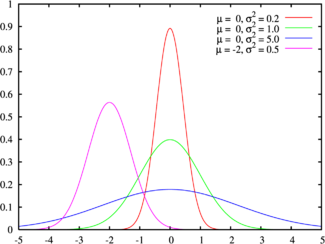有一点要注意某一点x的值f(x),并不是x点对应的概率值，他代表的是概率累积函数在这点的导数，也就是梯度，但是他其实可以近似的表示某个点落在高斯分布

x

∈

[

x

,

x

+

0.001

]

x\in[x,x+0.001]

所围城图形上的概率。  现在我们看一下高斯分布公式中的两个未知参数是怎么求解的，有没有感觉和前面求解硬币正面朝上的概率很熟悉，没错，它也是用极大似然估计求解的，只不过它有两个参数

u

,

σ

2

u,\sigma^{2}

，求解的套路是一样的，先求出高斯分布的对数似然函数，然后求导，令导数等于0，求解参数，这里要注意，由于有两个未知的参数，所以需要求偏导，令导数等于0，求解过程就不写了，直接列出最后的结果：

u

=

1

n

∑

i

=

1

n

x

i

u=\frac{1}{n}\sum_{i=1}^{n}x_{i}

σ

2

=

1

n

∑

i

=

1

n

(

x

i

−

u

)

2

\sigma^{2} = \frac{1}{n}\sum_{i=1}^{n}(x_{i}-u)^{2}

2，GMM求解
这里引用李航老师《统计学习方法》上的定义，如下：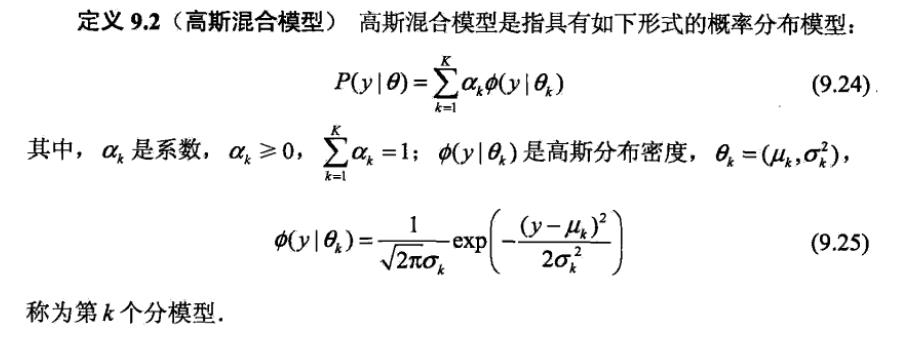GMM其实就是把多个高斯分布乘以一个系数

a

k

a_{k}

,然后在累加起来，有没有发现

a

k

a_{k}

和我们前面讲的EM估计中的向量k=[硬币1，硬币2，硬币1]很像，没有错它们都称为隐含变量，下面我们就用包含两个高斯分布的GMM来举例假设我们有10个身高数据

[

16

6

女

，

17

8

男

，

15

5

女

，

17

5

男

，

16

0

女

，

18

0

男

，

16

3

女

，

18

8

男

，

17

0

女

，

17

0

男

]

[166_{女}，178_{男}，155_{女}，175_{男}，160_{女}，180_{男}，163_{女}，188_{男}，170_{女}，170_{男}]

男生和女生的身高都分别符合一个高斯分布，根据前面的极大似然估计我们可以求出：

u

男

=

1

n

∑

i

=

1

n

x

i

=

1

5

(

178

+

175

+

180

+

188

+

170

)

=

178.2

u_{男}=\frac{1}{n}\sum_{i=1}^{n}x_{i} =\frac{1}{5}(178+175+180+188+170) = 178.2

σ

男

2

=

1

n

∑

i

=

1

n

(

x

i

−

u

)

2

=

1

5

(

(

178

−

178.5

)

2

+

(

175

−

178.5

)

2

+

(

180

−

178.5

)

2

+

(

188

−

178.5

)

2

+

(

170

−

178.5

)

2

)

=

44.2

\sigma^{2}_{男} = \frac{1}{n}\sum_{i=1}^{n}(x_{i}-u)^{2} =\frac{1}{5}((178-178.5)^{2}+(175-178.5)^{2}+(180-178.5)^{2}+\\ (188-178.5)^{2}+(170-178.5)^{2})=44.2

u

女

=

1

n

∑

i

=

1

n

x

i

=

1

5

(

166

+

155

+

160

+

163

+

170

)

=

162.8

u_{女}=\frac{1}{n}\sum_{i=1}^{n}x_{i}=\frac{1}{5}(166+155+160+163+170) = 162.8

σ

女

2

=

1

n

∑

i

=

1

n

(

x

i

−

u

)

2

=

1

5

(

(

166

−

162.8

)

2

+

(

155

−

162.8

)

2

+

(

160

−

162.8

)

2

+

(

163

−

162.8

)

2

+

(

170

−

162.8

)

2

)

=

32.7

\sigma^{2}_{女} = \frac{1}{n}\sum_{i=1}^{n}(x_{i}-u)^{2}=\frac{1}{5}((166-162.8)^{2}+(155-162.8)^{2}+(160-162.8)^{2}+\\ (163-162.8)^{2}+(170-162.8)^{2})=32.7

假设我们获得的10个身高数据是

[

16

6

未

知

，

17

8

未

知

，

15

5

未

知

，

17

5

未

知

，

16

0

未

知

，

18

0

未

知

，

16

3

未

知

，

18

8

未

知

，

17

0

未

知

，

17

0

未

知

]

[166_{未知}，178_{未知}，155_{未知}，175_{未知}，160_{未知}，180_{未知}，163_{未知}，188_{未知}，170_{未知}，170_{未知}]

，我们知道他们是属于两个高斯分布，我要如何求出这个高斯分布呢？，有没有一种熟悉的味道，和上面讲解的EM估计非常类似，其实他们的思想是一样的，我下面就一步一步计算一遍首先假设

u

男

u_{男}

=178，

σ

男

2

\sigma^{2}_{男}

=44，

u

女

u_{女}

=162，

σ

女

2

\sigma^{2}_{女}

=32，第一步，求第一个数据166的可能性为：

f

(

166

)

男

=

1

44

2

π

e

−

(

166

−

178

)

2

2

×

44

=

0.0735688

f(166)_{男}=\frac{1}{\sqrt{44}\sqrt{2\pi}}e^{-\frac{(166-178)^{2}}{2\times 44}}=0.0735688

f

(

166

)

女

=

1

32

2

π

e

−

(

166

−

162

)

2

2

×

32

=

0.345092

f(166)_{女}=\frac{1}{\sqrt{32}\sqrt{2\pi}}e^{-\frac{(166-162)^{2}}{2\times 32}}=0.345092

因为

f

(

166

)

男

<

f

(

166

)

女

f(166)_{男}<f(166)_{女}

,所以166这个数据属于女生的高斯分布的可能性大，下面我们计算剩余的数据；
身高男生高斯分布的可能性女生高斯分布的可能性1660.07356880.3450921780.3778830.008115791550.0009260940.2060641750.3411460.03160091600.009514020.416261800.3610910.002804741630.02930520.4362371880.1212940.0000111700.1826020.163011700.1826020.16301
我们根据可能性的大小，确定数据的属于那个分布，

16

6

女

，

17

8

男

，

15

5

女

，

17

5

男

，

16

0

女

，

18

0

男

，

16

3

女

，

18

8

男

，

17

0

男

，

17

0

男

166_{女}，178_{男}，155_{女}，175_{男}，160_{女}，180_{男}，163_{女}，188_{男}，170_{男}，170_{男}

第二步，根据极大似然估计来计算两个高斯分布的

u

,

σ

2

u,\sigma^{2}

第三步，判断新计算的

u

,

σ

2

u,\sigma^{2}

与上一次的

u

,

σ

2

u,\sigma^{2}

是否相等，如果不相等重复第一步和第二步；这里有个问题，我们知道GMM中有个权重系数

a

k

a_{k}

可是我们刚才并没有出现这个

a

k

a_{k}

，其实是为了方便直接使用男或女表示了，如果使用

a

k

a_{k}

表示，则数据

16

6

女

166_{女}

，就表示为

16

6

[

0

,

1

]

166_{[0,1]}

，GMM是多个高斯分布乘以系数

a

k

a_{k}

，然后相加得到的，所以：

16

6

女

=

16

6

[

0

,

1

]

=

0

×

男

生

高

斯

分

布

+

1

×

女

生

高

斯

分

布

=

女

生

高

斯

分

布

166_{女}=166_{[0,1]}=0\times 男生高斯分布+1\times女生高斯分布=女生高斯分布

共四种模式，用数字0~3来区分，激进程度与数值大小正相关。 0: Normal，1：low Bitrate， 2：Aggressive；3：Very Aggressive
2，帧长设置
3，高斯模型中特征向量选取
在WebRTC的VAD算法中用到了聚类的思想，只有两个类，一个类是语音，一个类是噪声，对每帧信号都求其是语音和噪声的概率，根据概率进行聚类，当然为了避免一帧带来的误差也有一个统计量判决在算法里，那么问题来了，选择什么样的特征作为高斯分布的输入呢?这关系到聚类结果的准确性，也即VAD性能，毋庸置疑，既然VAD目的是区分噪声和语音，那么噪声信号和语音信号这两种信号它们的什么特征相差最大呢?选择特征相差比较大自然能得到比较好的区分度。  众所周知，信号的处理分类主要有时域，频域和空域，从空域上看，webRTC的VAD是基于单麦克的，噪声和语音没有空间区分度的概念，在多麦克风场景，确实基于多麦克风的VAD算法，从时域上看，而者都是时变信号，且短时信号变化率比较小，所以推算来推算去只有频域的区分度可能是比较好的。  汽车噪声频谱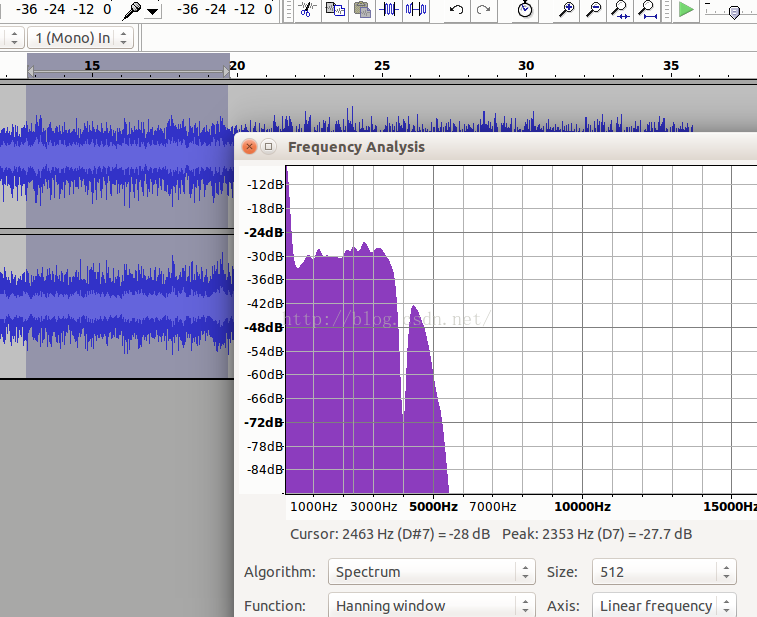粉红噪声频谱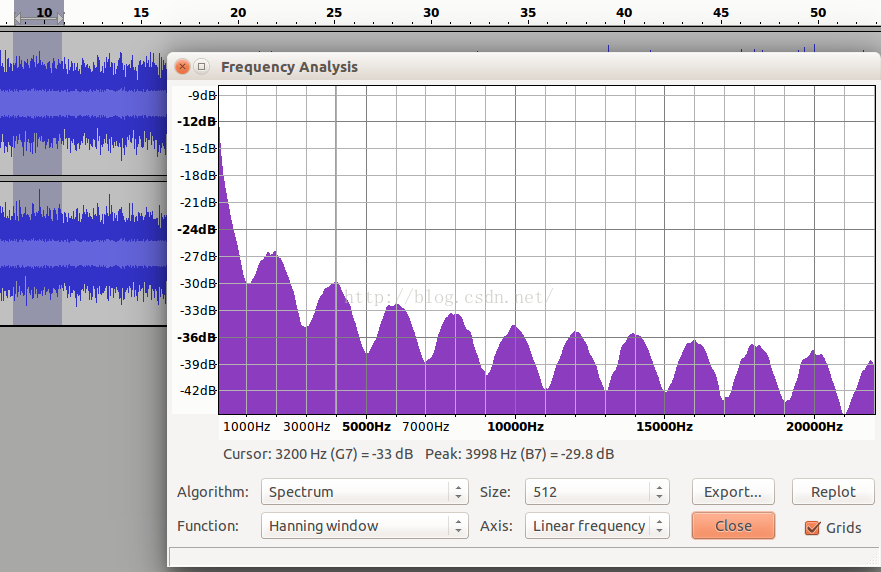白噪声频谱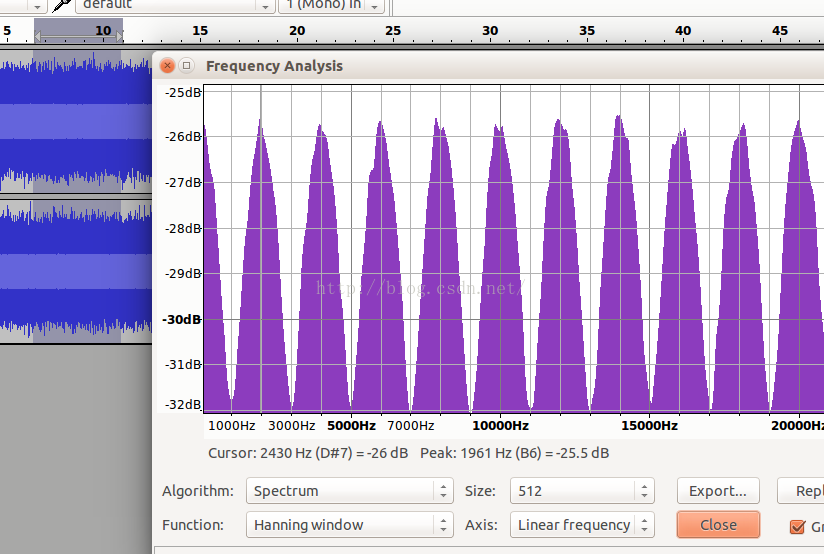语音声谱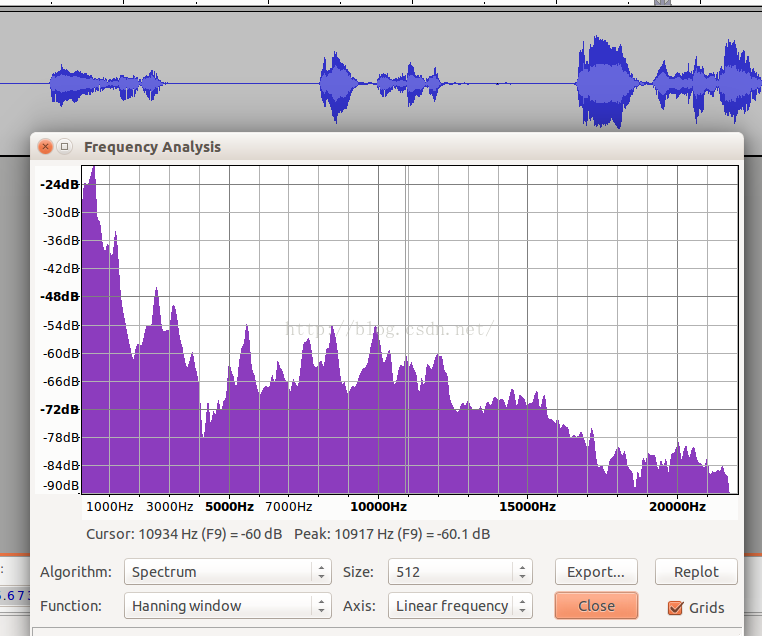从以上四个图中，可以看到从频谱来看噪声和语音，它们的频谱差异还是比较大，且以一个个波峰和波谷的形式呈现。  WebRTC正式基于这一假设，将频谱分成了6个子带。它们是：[80Hz,250Hz)，[250Hz,500Hz),[500Hz,1K),[1K,2K),[2K,3K),[3K,4K)。分别对应于feature,feature,feature,…,feature。  可以看到以1KHz为分界，向下500HZ，250Hz以及170HZ三个段，向上也有三个段，每个段是1KHz，这一频段涵盖了语音中绝大部分的信号能量，且能量越大的子带的区分度越细致。  我国交流电标准是220V~50Hz，电源50Hz的干扰会混入麦克风采集到的数据中且物理震动也会带来影响，所以取了80Hz以上的信号。  这个模型和我们上面讲个的身高的例子，原理是一样的，只是这个例子的输入不是身高的值，而是子带能量，这个GMM包含两个高斯分布，一个是语音的高斯分布，一个是噪音的高斯分布
3，计算流程
高斯模型有两个参数H0和H1，它们分表示的是噪声和语音，判决测试使用LRT（likelihood ratio test，似然比检测）。分为全局和局部两种情况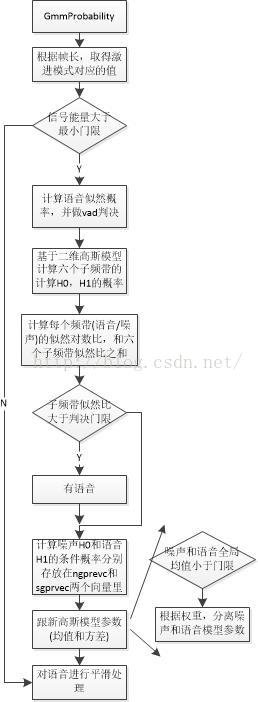4，参数更新
噪声均值更新，参数更新就是使用极大似然估计根据已经分类完的数据去重新计算均值

u

u

和方差

σ

2

\sigma^{2}

五，参考资料：
https://blog.csdn.net/shichaog/article/details/52399354/ https://www.jianshu.com/p/1121509ac1dc https://blog.csdn.net/zouxy09/article/details/8537620
展开全文千次阅读 2018-03-23 10:37:40
这几天对webrtc中的VAD算法做了一些研究，有了一些心得

但是这个GMM的具体情况是怎么样的，它的均值，方差，都是多少，使用特征的维度是多少？参数又是怎么更新的

一、GMM的 权重，均值，方差分析
频率域上分成了6个子带（channel），对每个子带求能量，初始值有一个GMM参数，包括 权重，均值，方差。
对噪声、语音，每个子带上的GMM的混合系数2，即2个高斯的mixture，一共6（6个channel，或者子带）*2（noise和speech）*2（每个GMM和混合系数是2）
你比如噪声的权重，kNoiseDataWeights，前六个和后六个分别是两个GMM的两组权重，Q7量化为2^7 =128
显然， 34/128 + 94/128 = 1; 62/128+66/128 = 1;...依次类推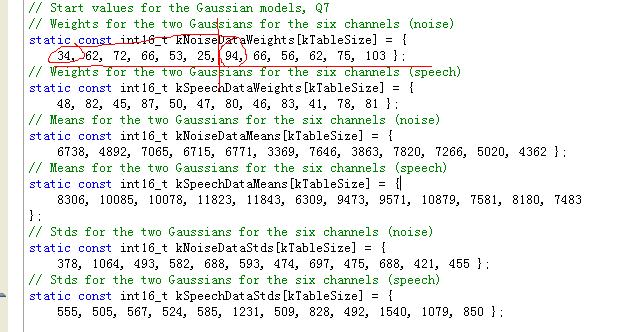使用的特征是6维的，但是这6维不是直接就使用一个GMM模型，而是裂解成6个子特征（6个channel），每个一维度的特征作为一个混合高斯模型的输入，每个高斯混合模型的混合系数是2
问题比较简单了，既然不是多维度的，那么协方差矩阵的 正定问题 就归在一个方差值上面，也就这一个方差数大于0即可。
二、高斯混合模型的后验概率
后验概率的公式我们先复习一下：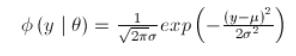（单维的公式就是简单明了啊）
1 / s 然后计算 1 / s^2 再  |tmp32| = (x - m)^2 / (2 * s^2), 再计算 exp(-(x - m)^2 / (2 * s^2))，最后算出来  (1 / s) * exp(-(x - m)^2 / (2 * s^2))
暂时还没有看出来 1/sqrt(2*pi) 在哪里，估计在后面，或者忽略了。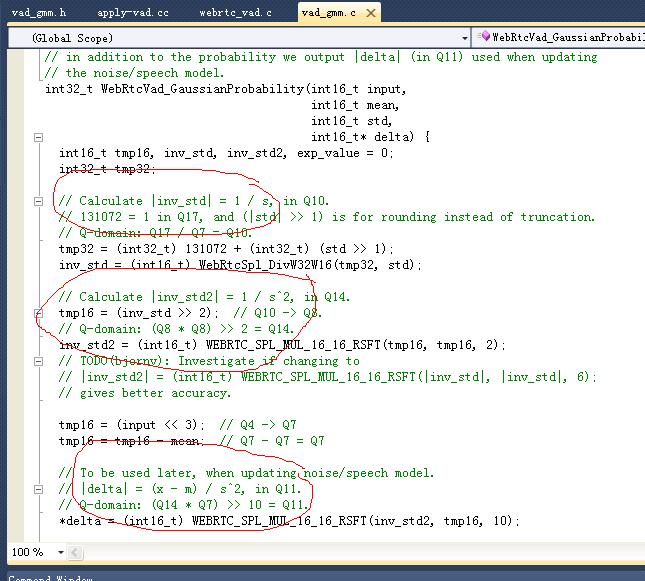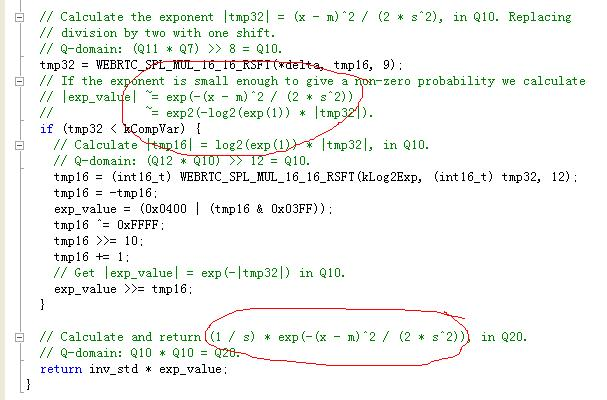注意输入的input是Q4的。实际上求后验概率，就这么简单，但是程序中非出来一个判断函数，这个主要是为了如果要求 x-> exp(-x)
当 |tmp32| = (x - m)^2 / (2 * s^2) （x是均值）这个值非常大的时候，超过一个范围 kCompVar = 22005的时候，认为 exp(-|tmp32|） 接近是 0直接给成0
这下面 令 x  = |tmp32| （下图中的红色的X）, 主要目的是使用了 ”换底公式” ，以前是求exp的幂，现在是求2的幂【2的幂在定点代码中比e的幂好算】
我们主要方向，x --- > exp(-x) 考虑到Q值，Q10的量 另外 log2(exp(1)) = 1.4427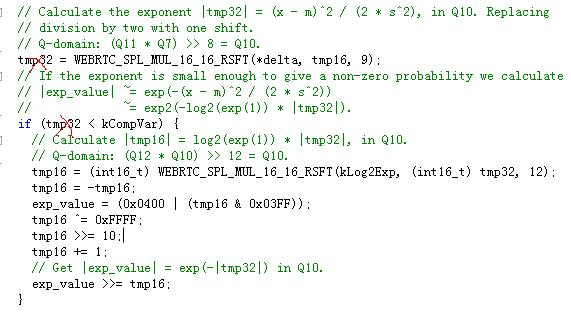x是Q10的，最后算出来的exp表达式也得是Q10，所以真正的 x到exp(-x)，应该是
exp(-x/1024)*1024 = 1024*( 2 ^ [log2(e))*(-x/1024)]) = 1024* 2^ (-1.4427* x/1024)
这里面用了一些技巧，主要是为了方便求2个幂，我们给这个表达式上下都乘以一个数，2^[fix(x*1.4427/1024)+1]
2^10 * 2^ (-1.4427* x/1024) = 2^ (10 + -1.4427*x/1024) = 2^(10 + -1.4427*x/1024 +1 + fix(x*1.4427 /1024) ) /2^[fix(x*1.4427/1024)+1]
乘以这个数的主要目的是为了使得  -1.4427*x/1024 + 1 + fix(x*1.4427 /1024) 能是一个不大于1的一个纯小数【小学概念】
上面那个除法表达式分子记为 Y(就是代码中的exp_value)，所以有 Y = 2^(10 + -1.4427*x/1024 + 1 + fix(x*1.4427 /1024) ),另外 分母记为2^[fix(x*1.4427/1024)+1]，最后分子准备右移（除法）就得结果了
对Y除2^10,                           Y/1024 = 2^(-1.4427*x/1024 + 1 + fix(x*1.4427 /1024) )

上面也说了，这个括号里面的数，应该为 1 + α ， α是一个接近0比较小的数，我们利用二项式展开公式，分解2的幂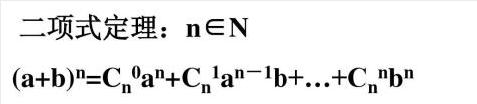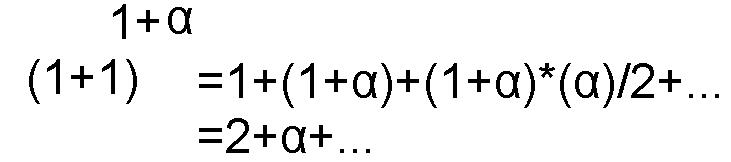Y/1024 ≈ 2 + -1.4427*x/1024 + fix(x*1.4427 /1024) 那么Y有
Y  = 1024 *（2 + fix(x*1.4427 /1024)-1.4427*x/1024）
= 1024* ( 1-x* 1.4427/1024+  fix( x * 1.4427 / 1024)+1)
=   1024- x * 1.4427+1024* fix( x * 1.4427 / 1024)+1024
=  2^16 - x * 1.4427 - 1024 *  (63) + 1024* fix( x * 1.4427 / 1024)  + 1024
=  2^16 - x * 1.4427 - 1024 *  (63 - fix( x * 1.4427 / 1024) ) + 1024
而         fix(( 2^16 - x * 1.4427) / 1024)=   63 - fix( x * 1.4427 / 1024); 【的确不是64减】
故  Y =     2^16 - x * 1.4427 -  1024*fix(( 2^16 - x * 1.4427) / 1024)  + 1024 = 2^16 - x * 1.4427-  1024*fix(( 2^16 - x * 1.4427) / 1024) + 1024
2^16 - x * 1.4427  是求相反数，代码中的刚从 WEB...MUL...RSFT算出来的 tmp16 = 1.4427* x ；
1024*fix(( 2^16 - x * 1.4427) / 1024) 为了抛掉整数，只留小数部分（我知道这个蓝色表达式求得是整数部分），所以前面要加个“-”
1024 加上1024
再来看代码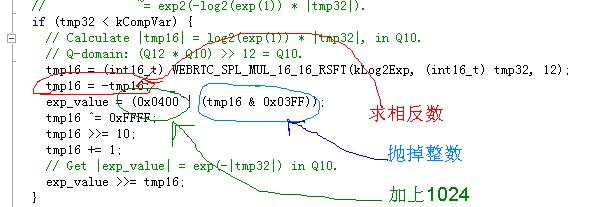分母就不用多说了：  2^[fix(x*1.4427/1024)+1]，除法(Y/tmp16)相当于右移 （exp_value>>tmp16）
22005 是这样算出来的   fix(22005*1.4427/1024)+1  = 32（最大右移数） ，那么 31 * 1024 /1.4427 =  2.2003e+004（倒算出来的，先有32-1）
Tips: 这个整体思路是要计算 x 到 exp(-x/1024)*1024,其中tmp16的值为 tmp16（最终值） = 2^(fix(x*1.4427/1024)+1)
我已经计算出来了 exp(-x/1024)*1024 = Y/tmp16 （令Y为分子，tmp16为分母）
我先计算Y/1024, 为的是这个数比较能用2的幂级数展开近似求，求出来之后, 得到Y，一个除法（Y/tmp16）就是最终结果
代码中为什么是 exp_value >> = tmp16?  它相当于 是在求一个除法，Y >>fix(x*1.4427/1024)+1
也就是 Y / 2^[fix(x*1.4427/1024)+1]
为什么exp的算法最后是个除法？原因是用了“换底公式”都换成2的幂去计算了

三、高斯混合模型的参数更新
参数更新问题，很多博客已经给出了很详细的说明，比如http://blog.csdn.net/shichaog/article/details/52399354  【这里感谢博主】
噪声均值的更新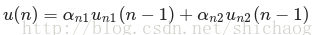参考代码 vad_sp.c中的  WebRtcVad_FindMinimum 函数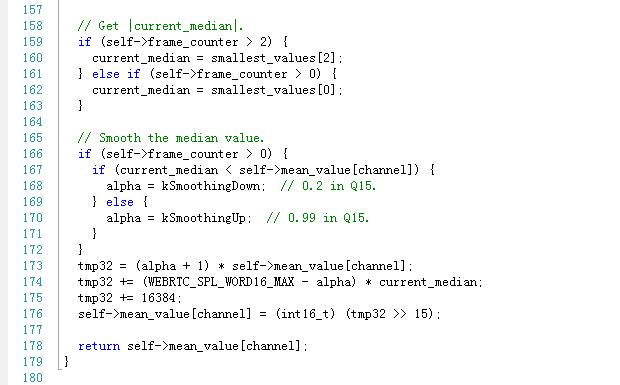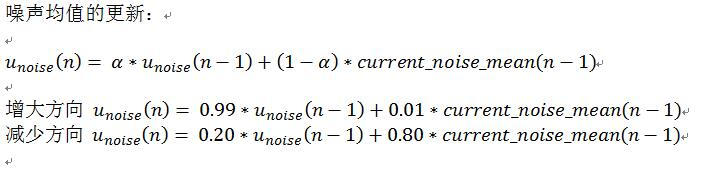从这个公式我们就可以看出来了，对于noise的mean增大，比较抠门，尽可能的保持原有的噪声水平不变
对缩小，放的比较开，这是为了保证噪声跟进能比较准确

对于下面的参数更新，我这里做一些啰嗦的说明，以便大家理解的更好一些，【先放成果照片，拜大神，后面我再详细说明】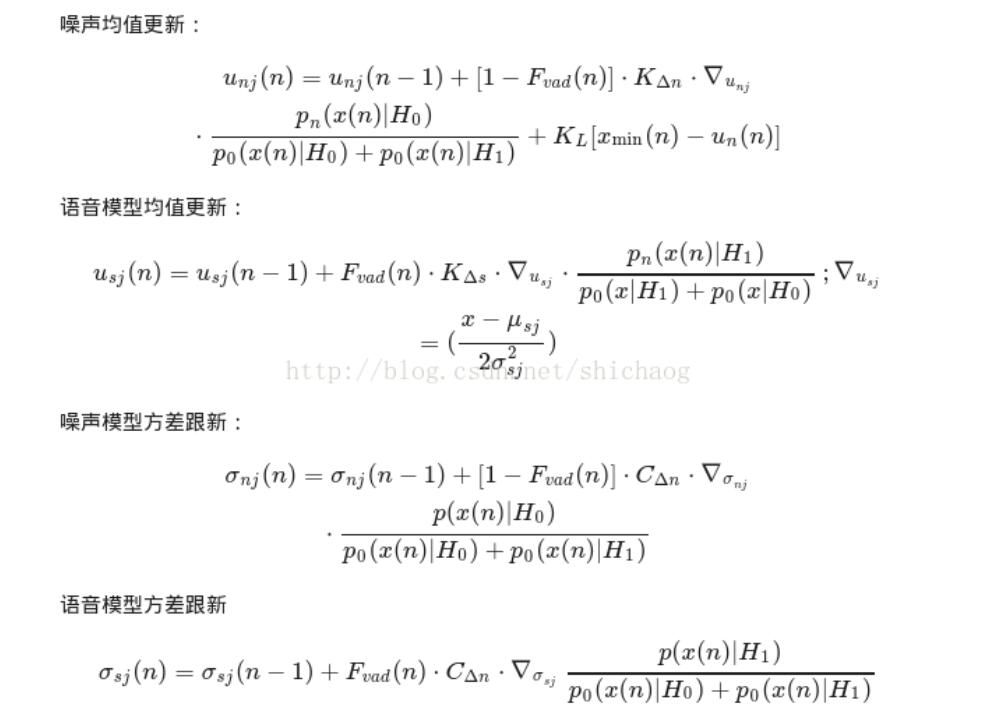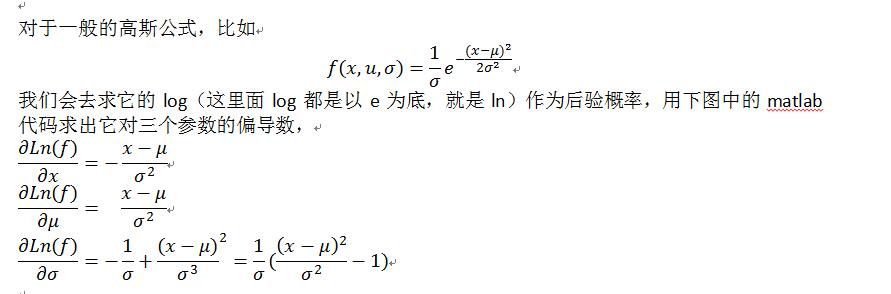偏导公式太复杂了，我也记不住，用matlab来算吧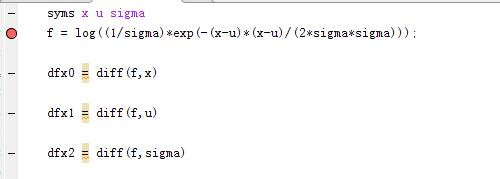代码最核心的更新部分：GmmProbability函数中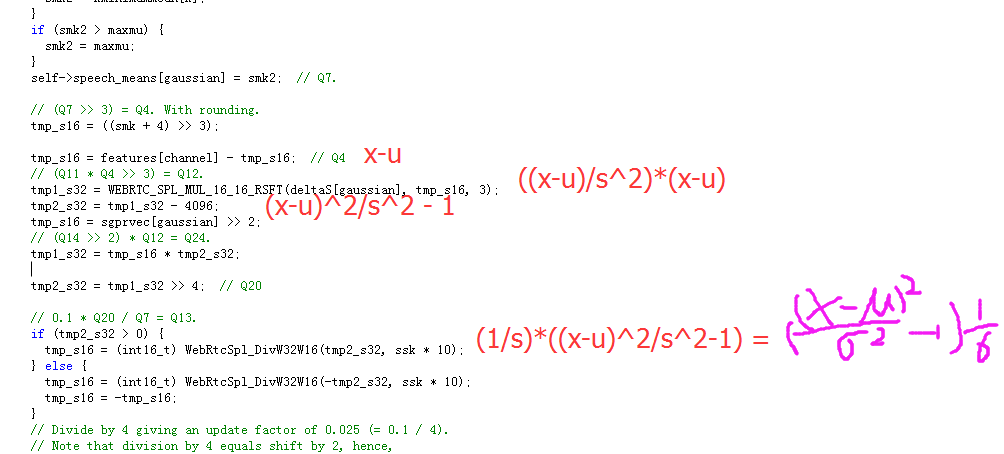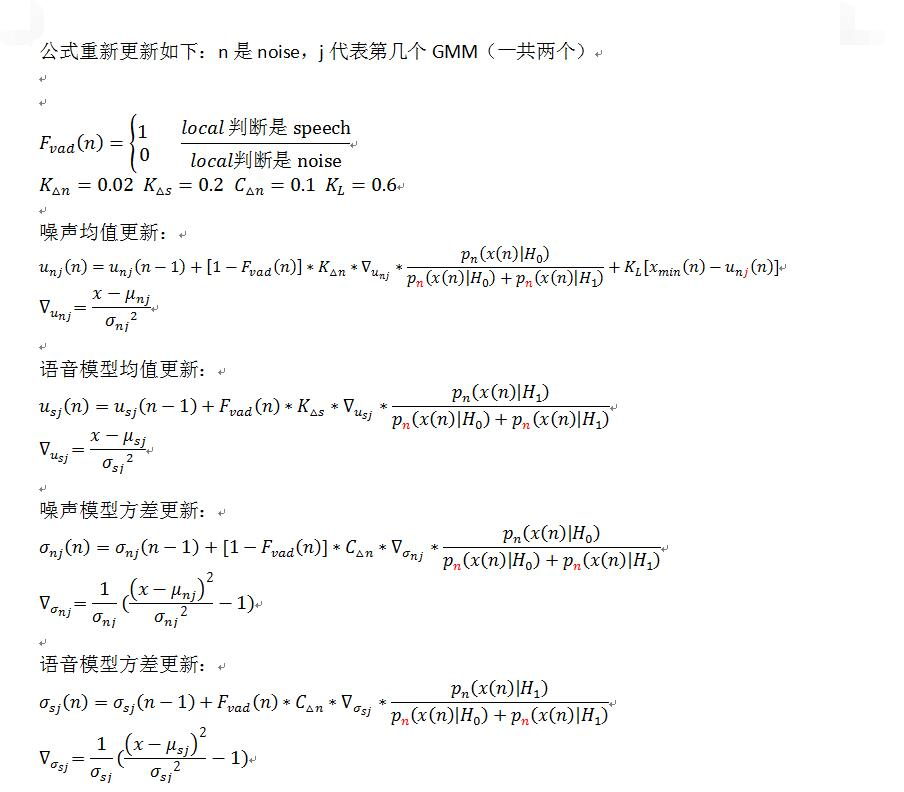展开全文技术

万次阅读 多人点赞 2016-09-01 14:40:04

我的书：购买链接：
京东购买链接
淘宝购买链接
当当购买链接
1.    可以成功检测到最低能量的语音(灵敏度)。 2.    如何在多噪环境下成功检测（漏检率和虚检率）。 漏检反应的是原本是语音但是没有检测出来，而虚检率反应的是不是语音信号而被检测成语音信号的概率。相对而言漏检是不可接受的，而虚检可以通过后端的ASR和NLP算法进一步过滤，但是虚检会带来系统资源利用率上升，随之系统的功耗和发热会进一步增加，而这会上升为可移动和随声携带设备的一个难题。 本文基于WebRTC的AEC算法，WebRTC的VAD模型采用了高斯模型，这一模型应用极其广泛。
高斯分布
高斯分布又称为正态分布（Normal distribution/Gaussian distribution）。 若随机变量X服从一个数学期望为μ，标准差为σ^2的高斯分布，则： X~N(μ，σ^2) 其概率密度函数为： f(x)=1/(√2π σ) e^(-〖(x-u)〗^2/(2σ^2 )) 高斯在webRTC中的使用： f(x_k |Z，r_k)=1/√2π e^(-(x_k-u_z )^2/(2σ^2 )) x_k是选取的特征向量，webRTC中指x_k是六个子带的能量（子带是80~250Hz，250~500Hz，500Hz~1K， 1~2K，2~3K，3~4KHz，变量feature_vector存放的就是子带能量序列），r_k是均值u_z和方差σ的参数结合，这两个参数决定了高斯分布的概率。Z=0情况是计算噪声的概率，Z=1是计算是语音的概率。
这里采用最高频率是4KHz的原因是，webRTC中程序将输入（48KHz，32HKz，16KHz）都下采样到8KHz，这样根据奎斯特频率定理，有用的频谱就是4KHz以下。

Webrtc采用的是GMM模型
等待视频链接地址。
WebRTC算法流程
共四种模式，用数字0~3来区分，激进程度与数值大小正相关。 0: Normal，1：low Bitrate， 2：Aggressive；3：Very Aggressive 这些激进模式是和以下参数是息息相关的。
<comman_audio/vad/vad_core.c>
// Mode 0, Quality.
static const int16_t kOverHangMax1Q = { 8, 4, 3 };
static const int16_t kOverHangMax2Q = { 14, 7, 5 };
static const int16_t kLocalThresholdQ = { 24, 21, 24 };
static const int16_t kGlobalThresholdQ = { 57, 48, 57 };
// Mode 1, Low bitrate.
static const int16_t kOverHangMax1LBR = { 8, 4, 3 };
static const int16_t kOverHangMax2LBR = { 14, 7, 5 };
static const int16_t kLocalThresholdLBR = { 37, 32, 37 };
static const int16_t kGlobalThresholdLBR = { 100, 80, 100 };
// Mode 2, Aggressive.
static const int16_t kOverHangMax1AGG = { 6, 3, 2 };
static const int16_t kOverHangMax2AGG = { 9, 5, 3 };
static const int16_t kLocalThresholdAGG = { 82, 78, 82 };
static const int16_t kGlobalThresholdAGG = { 285, 260, 285 };
// Mode 3, Very aggressive.
static const int16_t kOverHangMax1VAG = { 6, 3, 2 };
static const int16_t kOverHangMax2VAG = { 9, 5, 3 };
static const int16_t kLocalThresholdVAG = { 94, 94, 94 };
static const int16_t kGlobalThresholdVAG = { 1100, 1050, 1100 };
它们在计算高斯模型概率时用到。
2帧长设置
3 高斯模型中特征向量选取
在WebRTC的VAD算法中用到了聚类的思想，只有两个类，一个类是语音，一个类是噪声，对每帧信号都求其是语音和噪声的概率，根据概率进行聚类，当然为了避免一帧带来的误差也有一个统计量判决在算法里，那么问题来了，选择什么样的特征作为高斯分布的输入呢?这关系到聚类结果的准确性，也即VAD性能，毋庸置疑，既然VAD目的是区分噪声和语音，那么噪声信号和语音信号这两种信号它们的什么特征相差最大呢?选择特征相差比较大自然能得到比较好的区分度。   众所周知，信号的处理分类主要有时域，频域和空域，从空域上看，webRTC的VAD是基于单麦克的，噪声和语音没有空间区分度的概念，在多麦克风场景，确实基于多麦克风的VAD算法，从时域上看，而者都是时变信号，且短时信号变化率比较小，所以推算来推算去只有频域的区分度可能是比较好的。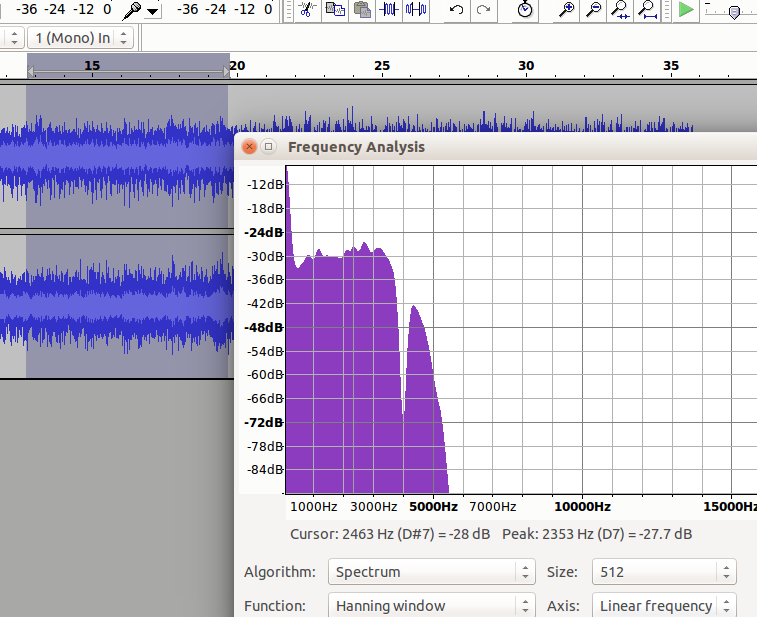汽车噪声频谱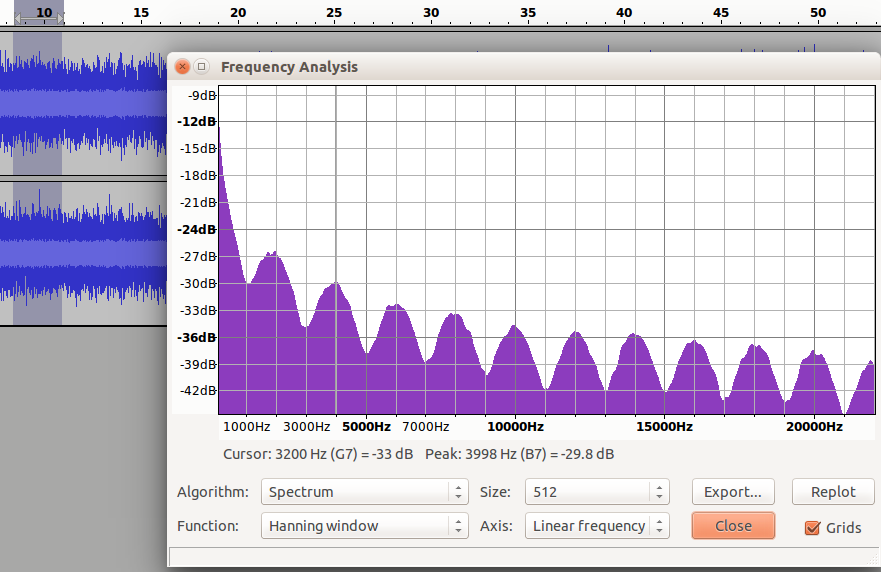粉红噪声频谱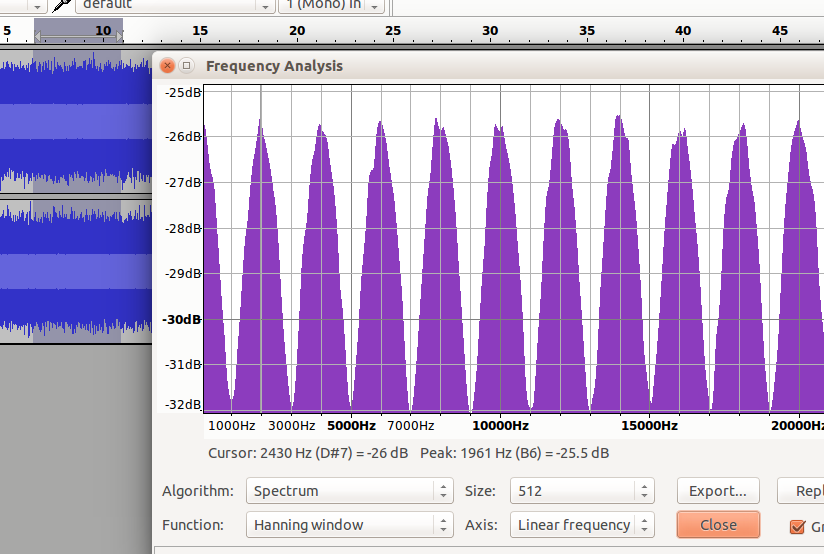白噪声频谱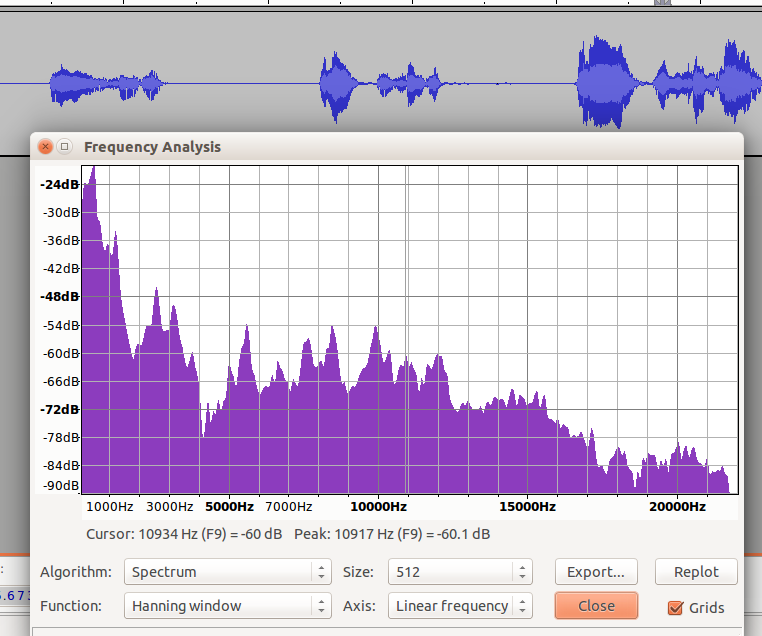语音声谱
从以上四个图中，可以看到从频谱来看噪声和语音，它们的频谱差异还是比较大，且以一个个波峰和波谷的形式呈现。   WebRTC正式基于这一假设，将频谱分成了6个子带。它们是：     80Hz~250Hz，250Hz~500Hz,500Hz~1K,1K~2K,2K~3K,3K~4K。分别对应于feature,feature,feature,...,feature。 可以看到以1KHz为分界，向下500HZ，250Hz以及170HZ三个段，向上也有三个段，每个段是1KHz，这一频段涵盖了语音中绝大部分的信号能量，且能量越大的子带的区分度越细致。   我国交流电标准是220V~50Hz，电源50Hz的干扰会混入麦克风采集到的数据中且物理震动也会带来影响，所以取了80Hz以上的信号。
在webRTC计算的函数在filter_bank.c文件中，前面说的基于激活的DNN也可以是基于fbank特征。
高通滤波器设计
高通滤波器的作用有两点：1.滤除直流分量，2提升高频成分（人耳对3.5KHz最为敏感）
// High pass filtering, with a cut-off frequency at 80 Hz, if the |data_in| is
// sampled at 500 Hz.
//
// - data_in      [i]   : Input audio data sampled at 500 Hz.
// - data_length  [i]   : Length of input and output data.
// - filter_state [i/o] : State of the filter.
// - data_out     [o]   : Output audio data in the frequency interval
//                        80 - 250 Hz.
static void HighPassFilter(const int16_t* data_in, size_t data_length,
int16_t* filter_state, int16_t* data_out) {
size_t i;
const int16_t* in_ptr = data_in;
int16_t* out_ptr = data_out;
int32_t tmp32 = 0;

// The sum of the absolute values of the impulse response:
// The zero/pole-filter has a max amplification of a single sample of: 1.4546
// Impulse response: 0.4047 -0.6179 -0.0266  0.1993  0.1035  -0.0194
// The all-zero section has a max amplification of a single sample of: 1.6189
// Impulse response: 0.4047 -0.8094  0.4047  0       0        0
// The all-pole section has a max amplification of a single sample of: 1.9931
// Impulse response: 1.0000  0.4734 -0.1189 -0.2187 -0.0627   0.04532

for (i = 0; i < data_length; i++) {
// All-zero section (filter coefficients in Q14).
tmp32 = kHpZeroCoefs * *in_ptr;
tmp32 += kHpZeroCoefs * filter_state;
tmp32 += kHpZeroCoefs * filter_state;
filter_state = filter_state;
filter_state = *in_ptr++;

// All-pole section (filter coefficients in Q14).
tmp32 -= kHpPoleCoefs * filter_state;
tmp32 -= kHpPoleCoefs * filter_state;
filter_state = filter_state;
filter_state = (int16_t) (tmp32 >> 14);
*out_ptr++ = filter_state;
}
}

WebRTC在设计该滤波器上还是很有技巧的，技巧有二： 1.    定点数计算，指两个方面，一是滤波系数量化，而是计算过程的定点化，高斯模型计算也使用了这一技巧。 2.    舍入技巧，减少运算量。 下面就来看看，这些技巧是如何使用的，首先根据代码的注释可以看出， 全零点和全极点脉冲响应的实际上是浮点数，它们脉冲响应分别是： 0.4047 -0.8094  0.4047  0       0        0 1.0000  0.4734 -0.1189 -0.2187 -0.0627   0.04532 所以可见应该是六阶方程，但是超过3阶后，零点全零，极点数值较小，这时适当增大第三个数值，达到减少计算次数的目的。
量化是按照2的十四次方进行定点化。这是因为最差情况下，零极点的放大倍数不超过两倍，所以16位数可以表示的下来。其零极点绘图如下：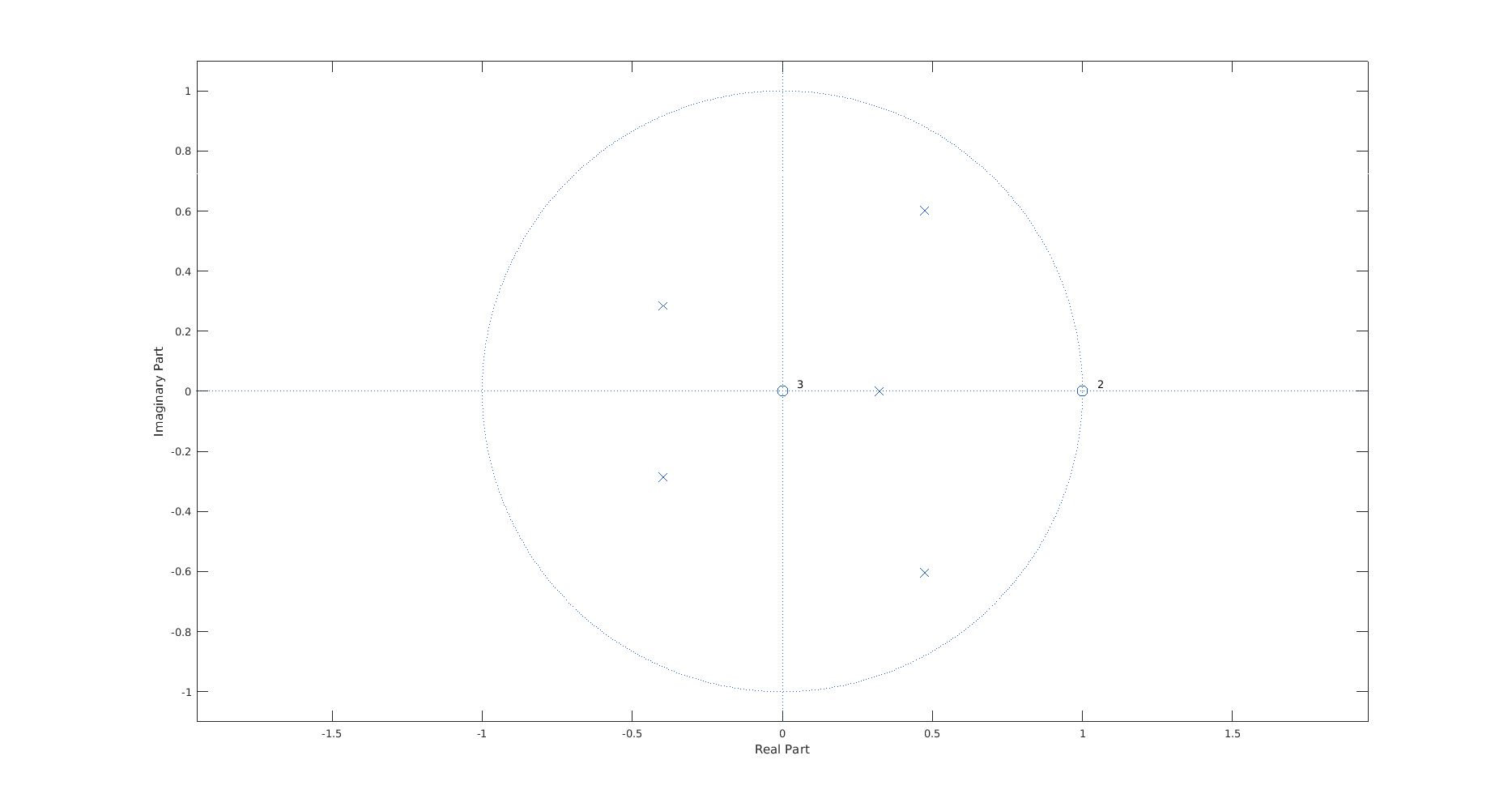对这两个图的解释就忽略了，能够看懂上述代码和两张图的意义，就可以更改滤波器的特性了，对不要相位信息的，采用IIR比FIR达到相同的增益平坦度需要的阶数要少。关于高通滤波器的设计还有疑问的可以留言共同交流。频响如下：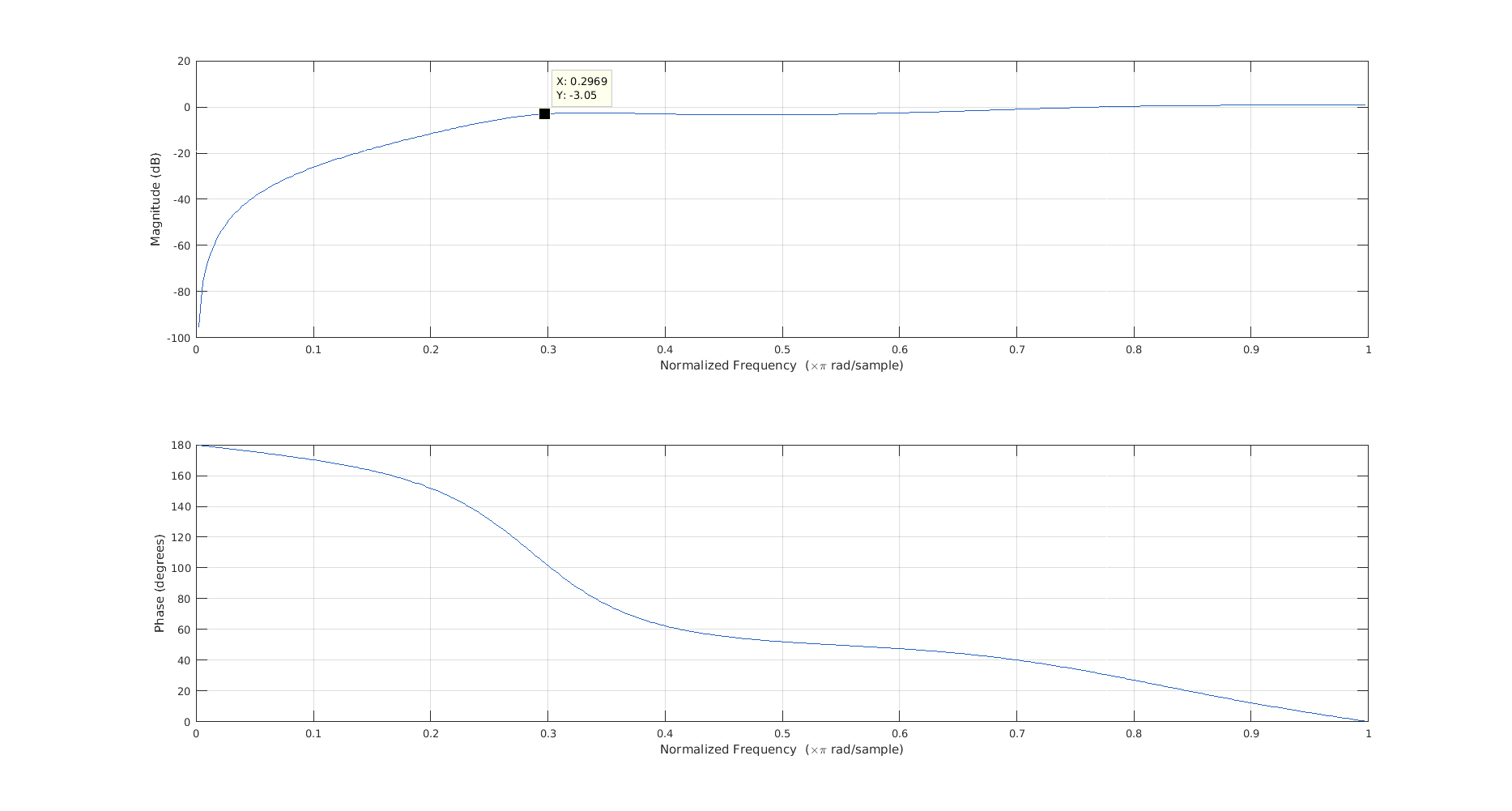int WebRtcVad_CalcVad8khz(VadInstT* inst, const int16_t* speech_frame,
size_t frame_length)
{
int16_t feature_vector[kNumChannels], total_power;

// Get power in the bands
feature_vector);

inst->vad = GmmProbability(inst, feature_vector, total_power, frame_length);

}

计算流程
高斯模型有两个参数H0和H1，它们分表示的是噪声和语音，判决测试使用LRT（likelihood ratio test）。分为全局和局部两种情况。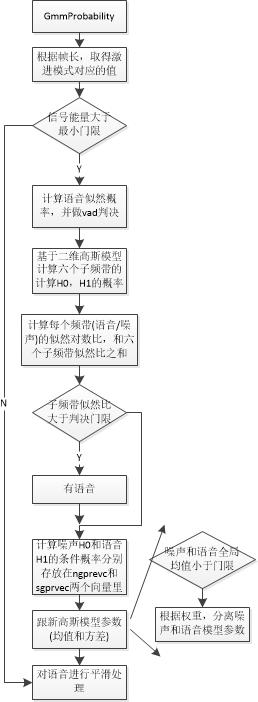a)高斯概率计算采用的高斯公式如下：
这里其实采用了两个参数高斯分布，但是假设了这两个参数是相互独立的：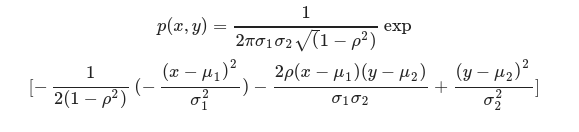这里要做个简化以减少计算量。将这两个高斯看成是不相关的，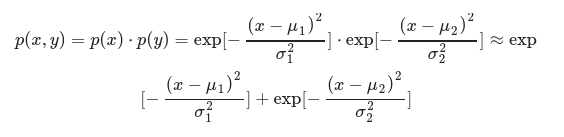这里可以看到做了三个简化，第一个是把指数前的系数省掉了，这是因为在做似然比检验时，可以消掉，第二个简化是假设这两个高斯分布是不相关的，第三个简化是将乘法近似化简成加法。使用高斯分布这个在kaldi中也有例子，kaldi中基于GMM的语言模型分成三种，第一种是类似这里的独立同分布模型，第二种是互相关对角阵元素非零，第三种是全高斯互相关模型。
用多个高斯来近似一个语音包络这种方法，在插值时也用到，即用多个sinc函数进行分数位插值。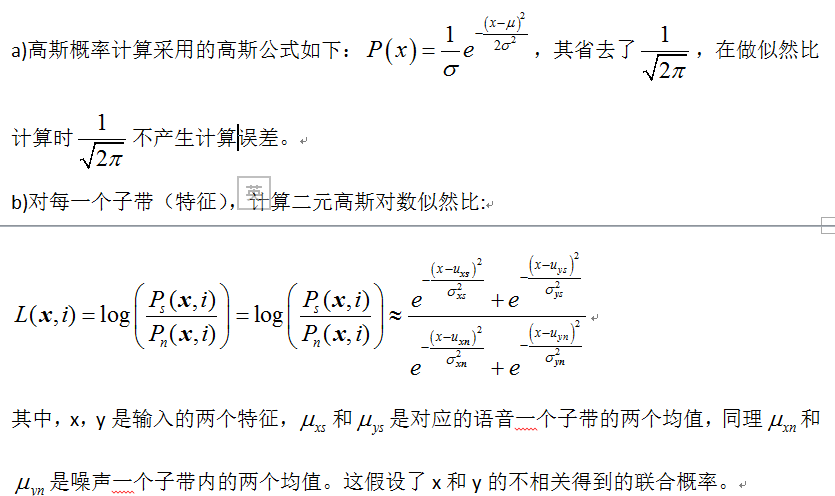c)对数似然比，分为全局和局部，全局是六个子带之加权之和，而局部是指每一个子带则是局部，所以语音判决会先判断子带，子带判断没有时会判断全局，只要有一方过了，就算有语音，公式表达如下：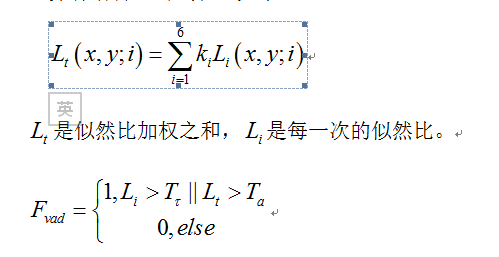后记：
// Spectrum Weighting
static const int16_t kSpectrumWeight[kNumChannels] = { 6, 8, 10, 12, 14, 16 };
static const int16_t kNoiseUpdateConst = 655; // Q15
static const int16_t kSpeechUpdateConst = 6554; // Q15
static const int16_t kBackEta = 154; // Q8
// Minimum difference between the two models, Q5
static const int16_t kMinimumDifference[kNumChannels] = {
544, 544, 576, 576, 576, 576 };
// Upper limit of mean value for speech model, Q7
static const int16_t kMaximumSpeech[kNumChannels] = {
11392, 11392, 11520, 11520, 11520, 11520 };
// Minimum value for mean value
static const int16_t kMinimumMean[kNumGaussians] = { 640, 768 };
// Upper limit of mean value for noise model, Q7
static const int16_t kMaximumNoise[kNumChannels] = {
9216, 9088, 8960, 8832, 8704, 8576 };
// Start values for the Gaussian models, Q7
// Weights for the two Gaussians for the six channels (noise)
static const int16_t kNoiseDataWeights[kTableSize] = {
34, 62, 72, 66, 53, 25, 94, 66, 56, 62, 75, 103 };
// Weights for the two Gaussians for the six channels (speech)
static const int16_t kSpeechDataWeights[kTableSize] = {
48, 82, 45, 87, 50, 47, 80, 46, 83, 41, 78, 81 };
// Means for the two Gaussians for the six channels (noise)
static const int16_t kNoiseDataMeans[kTableSize] = {
6738, 4892, 7065, 6715, 6771, 3369, 7646, 3863, 7820, 7266, 5020, 4362 };
// Means for the two Gaussians for the six channels (speech)
static const int16_t kSpeechDataMeans[kTableSize] = {
8306, 10085, 10078, 11823, 11843, 6309, 9473, 9571, 10879, 7581, 8180, 7483
};
// Stds for the two Gaussians for the six channels (noise)
static const int16_t kNoiseDataStds[kTableSize] = {
378, 1064, 493, 582, 688, 593, 474, 697, 475, 688, 421, 455 };
// Stds for the two Gaussians for the six channels (speech)
static const int16_t kSpeechDataStds[kTableSize] = {
555, 505, 567, 524, 585, 1231, 509, 828, 492, 1540, 1079, 850 };
参数更新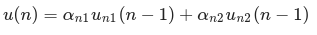模型参数跟新
跟新噪声均值，语音均值，噪声方差，语音方差，自适应也就体现在这里。
噪声跟新：只在非语音帧进行跟新，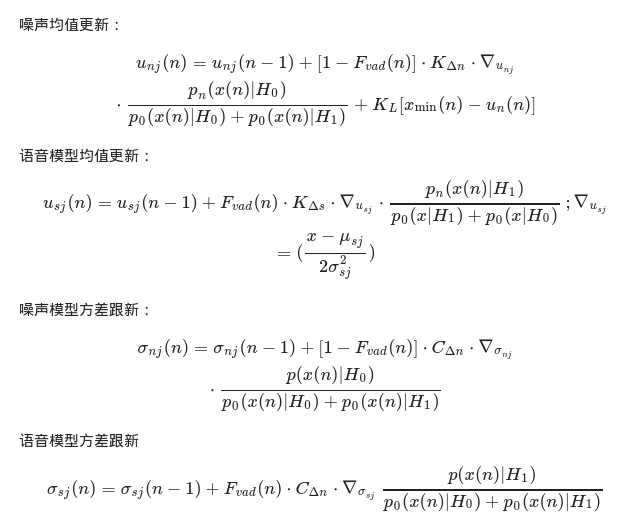另外：
现在也有基于DNN方法的进行VAD检测的，简单的检测使用两层LSTM外交BN（batch normalization）就可以实现这个功能。
展开全文语音活动检测算法大致可以分为三类，第一类就是最简单的基于阈值的判别方法，这个以前讲过了，参考语音活动检测；第二类是WebRTC使用的基于GMM的检测方法；第三类就是基于深度学习的检测方法，这个也讲过了，参考使用LSTM进行端点检测。废话不多说，开始进入正题。
Introduction
    enum Aggressiveness {        kVadNormal = 0,        kVadLowBitrate = 1,        kVadAggressive = 2,        kVadVeryAggressive = 3    };

Initialization
int WebRtcVad_InitCore(VadInstT *self) {
int i;

if (self == NULL) {
return -1;
}

// Initialization of general struct variables.
self->vad = 1;  // Speech active (=1).
self->frame_counter = 0;
self->over_hang = 0;
self->num_of_speech = 0;

// Initialization of downsampling filter state.
memset(self->downsampling_filter_states, 0,
sizeof(self->downsampling_filter_states));

// Initialization of 48 to 8 kHz downsampling.
WebRtcSpl_ResetResample48khzTo8khz(&self->state_48_to_8);

for (i = 0; i < kTableSize; i++) {
self->noise_means[i] = kNoiseDataMeans[i];
self->speech_means[i] = kSpeechDataMeans[i];
self->noise_stds[i] = kNoiseDataStds[i];
self->speech_stds[i] = kSpeechDataStds[i];
}

// Initialize Index and Minimum value vectors.
for (i = 0; i < 16 * kNumChannels; i++) {
self->low_value_vector[i] = 10000;
self->index_vector[i] = 0;
}

// Initialize splitting filter states.
memset(self->upper_state, 0, sizeof(self->upper_state));
memset(self->lower_state, 0, sizeof(self->lower_state));

// Initialize high pass filter states.
memset(self->hp_filter_state, 0, sizeof(self->hp_filter_state));

// Initialize mean value memory, for WebRtcVad_FindMinimum().
for (i = 0; i < kNumChannels; i++) {
self->mean_value[i] = 1600;
}

// Set aggressiveness mode to default (=|kDefaultMode|).
if (WebRtcVad_set_mode_core(self, kDefaultMode) != 0) {
return -1;
}

self->init_flag = kInitCheck;

return 0;
}

Updation
计算局部(每个子带)语音和噪声的概率用于更新GMM参数。  跟踪每个子带特征的最小值(WebRtcVad_FindMinimum), 函数对每个特征求出了100个帧里头的16个最小值。这些最小值都有一个age，最大不超过100，如果当前特征是100 帧里 16 个最小值之一，那么计算并返回五个最小值的中位数。这里得到的最小值后面会用来更新噪声。  更新GMM参数即语音/噪声的均值和方差，其中噪声的均值只在当前语音帧为非语音帧时进行更新。  当语音高斯模型和噪声高斯模型很相似时，分离它们。
Conclusion

本文相关代码，在公众号语音算法组菜单栏点击Code获取。

参考文献：
.  实时语音处理实践指南
.  A Statistical Model-Based Voice Activity Detection
展开全文语音信号处理filterbank

千次阅读 多人点赞 2018-11-21 21:11:48
• 图像特征检测算法-SIFT的Python实现，下载的文件为vlfeat-0.9.20-bin.tar.gz，解压缩后，将vlfeat-0.9.20/bin/win64文件夹下的sift.exe和vl.dll拷贝到当前工作目录下。
• WebRTC 音频算法 附完整C代码 WebRTC提供一套音频处理引擎， 包含以下算法： AGC自动增益控制(Automatic Gain Control) ANS噪音抑制(Automatic Noise Suppression) AEC是声学回声消除(Acoustic Echo Canceller ...
• stds)和噪声方差(noise_stds) 代码 import collections import contextlib import sys import wave import webrtcvad import shutil def read_wave(filename): path = filename with contextlib.closing(wave.open...
• ## Webrtc AGC 算法原理介绍（六）

千次阅读 热门讨论 2017-02-06 10:05:41
Webrtc AGC 算法原理介绍（六）零、前言本系列介绍Webrtc的agc算法webrtc的agc算法对各种情况作了较为详尽的考虑，而且使用了的定点数的方法来实现，因此内容比较多。尽量在这几篇文章中描述清楚。自动增益控制
• ## Webrtc AGC 算法原理介绍（一）

万次阅读 多人点赞 2016-09-19 19:03:52
Webrtc AGC 算法原理介绍（一）标签（空格分隔）： 音频算法零、前言本系列介绍Webrtc的agc算法webrtc的agc算法对各种情况作了较为详尽的考虑，而且使用了的定点数的方法来实现，因此内容比较多。尽量再这几篇文章...自动增益控制
• Webrtc AGC 算法原理介绍（四）零、前言本系列介绍Webrtc的agc算法webrtc的agc算法对各种情况作了较为详尽的考虑，而且使用了的定点数的方法来实现，因此内容比较多。尽量在这几篇文章中描述清楚。一、WebRtcAgc_...自动增益控制自动增益控制
• Webrtc AGC 算法原理介绍（五）零、前言本系列介绍Webrtc的agc算法webrtc的agc算法对各种情况作了较为详尽的考虑，而且使用了的定点数的方法来实现，因此内容比较多。尽量在这几篇文章中描述清楚。一、WebRtcAgc_...自动增益控制
• WEBRTC AGC 算法原理初识（一）1、AGC 初识2、WEBRTC 的 AGC算法3、主要配置4、主要接口 1、AGC 初识     自动增益控制电路的作用是：当输入信号电压变化很大时，保持接收机输出电压恒定或基本不变。具体地说，当...
• 音频算法零、前言本系列介绍Webrtc的agc算法webrtc的agc算法对各种情况作了较为详尽的考虑，而且使用了的定点数的方法来实现，因此内容比较多。尽量在这几篇文章中描述清楚。自动增益控制 信号处理
• 本系列介绍Webrtc的agc算法webrtc的agc算法对各种情况作了较为详尽的考虑，而且使用了的定点数的方法来实现，因此内容比较多。尽量在这几篇文章中描述清楚。 一、AGC的三个流程 函数 调用 功能 ...
• 本系列介绍Webrtc的agc算法webrtc的agc算法对各种情况作了较为详尽的考虑，而且使用了的定点数的方法来实现，因此内容比较多。尽量在这几篇文章中描述清楚。 一、WebRtcAgc_ProcessAnalog WebRtcAgc_ProcessAnalog...
• 本系列介绍Webrtc的agc算法webrtc的agc算法对各种情况作了较为详尽的考虑，而且使用了的定点数的方法来实现，因此内容比较多。尽量在这几篇文章中描述清楚。 一、WebRtcAgc_ProcessDigital ProcessDigital是AGC的...
• WebRTC提供一套音频处理引擎， 包含以下算法： AGC自动增益控制(Automatic Gain Control) ANS噪音抑制(Automatic Noise Suppression) AEC是声学回声消除(Acoustic Echo Canceller for Mobile) VAD是静音检测...
• webrtc的回声抵消(aec、aecm)算法主要包括以下几个重要模块： 回声时延估计 NLMS(归一化最小均方自适应算法) NLP（非线性滤波） CNG(舒适噪声产生） 回声时延估计 这张图很多东西可以无视，我们重点...
• webrtc的agc算法对各种情况作了较为详尽的考虑，而且使用了的定点数的方法来实现，因此内容比较多。尽量再这几篇文章中描述清楚。 一、AGC模块的结构与简介 较新的webrtc已经把原来的agc模块移动到了一个叫做......